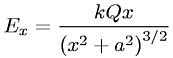Equations > Physics > Electricity and Magnetism > Electric field on the axis of a ring charge

### Electric field on the axis of a ring chargeLatex Code:

MathML Code:

 ${E}_{x}=\frac\mathrm{kQx}{\left({x}^{2}+{a}^{2}\right)}^{3\mathord/\vphantom322}$

MathType 5.0: